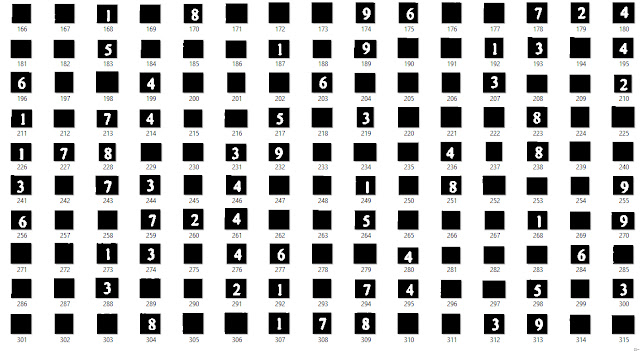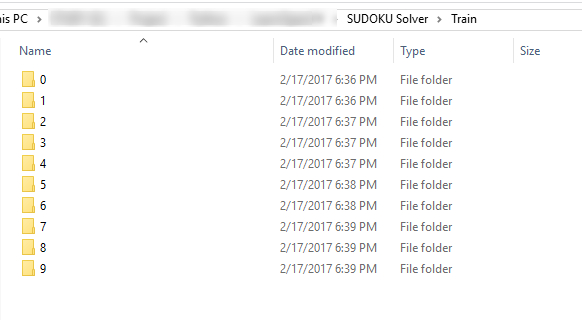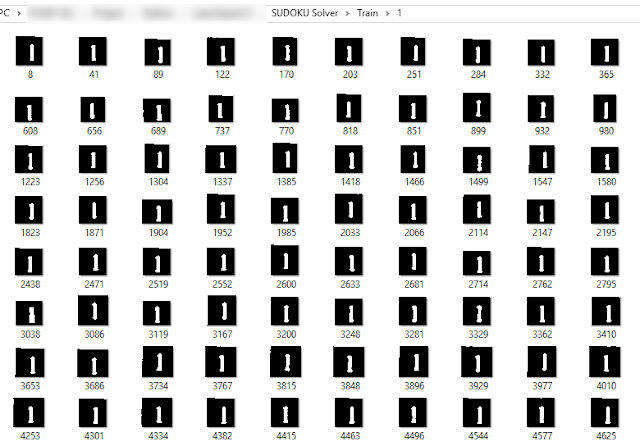# Building a simple SUDOKU Solver from scratch - Part 2: Digit Number Recognition using SVM

In this post, we will continue the previous tutorial on recognizing digital number were extracted from SUDOKU board. See part 1
In part 1 of this series, we learned how to detect lines and extract the digit numbers from the SUDOKU board. It’s easy to extract digit numbers and save it as images (Uncomment the code from Part 1 to save your own digit blocks). Now, let’s talk about training those extracted digital numbers with SVM

With the code from Part 1, we can easily save more than 1,000 digit images within a second.Fig. 1. The extracted digit images

To train the dataset with a supervised machine learning method like SVM, we need to label the dataset beforehand. With this dataset, we have 10 labels (from 0 -> 9). So, the idea is that we will create 10 folders with the name from 0 to 9 and put those images into the corresponding folder.
It’s time-consuming because we have a lot of images :(
Don’t worry! I already created a labeled dataset for you. Check out my GitHub repository to download the dataset: https://github.com/huuquan1994/Sudoku-Solver
I divided it into 2 parts, training set, and testing set. 5000 images in the training set (500 images in each folder) and ~3000 test images in the testing set.Fig. 2. We need to create 10 folders to store the imagesFig. 3. Folder with the images labeled "1"

We have all we need! Now, let’s train SVM to recognize digital number. We will use LinearSVM in Sklearn to train the dataset, using joblib to save the SVM model after training. For training, first, we resize the size of each training image to $$36\times36$$. After that, we train those resized images with LinearSVM. You can see the code below

import numpy as np
from sklearn.svm import LinearSVC
import os
import cv2
import joblib

# Generate training set
TRAIN_PATH = "Dataset\Train"
list_folder = os.listdir(TRAIN_PATH)
trainset = []
for folder in list_folder:
flist = os.listdir(os.path.join(TRAIN_PATH, folder))
for f in flist:
im = cv2.cvtColor(im, cv2.COLOR_RGB2GRAY )
im = cv2.resize(im, (36,36))
trainset.append(im)
# Labeling for trainset
train_label = []
for i in range(0,10):
temp = 500*[i]
train_label += temp

# Generate testing set
TEST_PATH = "Dataset\Test"
list_folder = os.listdir(TEST_PATH)
testset = []
test_label = []
for folder in list_folder:
flist = os.listdir(os.path.join(TEST_PATH, folder))
for f in flist:
im = cv2.cvtColor(im, cv2.COLOR_RGB2GRAY )
im = cv2.resize(im, (36,36))
testset.append(im)
test_label.append(int(folder))
trainset = np.reshape(trainset, (5000, -1))

# Create an linear SVM object
clf = LinearSVC()

# Perform the training
clf.fit(trainset, train_label)
print("Training finished successfully")

# Testing
testset = np.reshape(testset, (len(testset), -1))
y = clf.predict(testset)
print("Testing accuracy: " + str(clf.score(testset, test_label)))

joblib.dump(clf, "classifier.pkl", compress=3)


After training, we will have an SVM model named: classifier.pkl.
We can use this file to recognize block images.Fig. 4. Result of recoginited digits from SVM

See this code for more details:

import cv2
import numpy as np
import joblib

font = cv2.FONT_HERSHEY_SIMPLEX
ratio2 = 3
kernel_size = 3
lowThreshold = 30

is_print = True

cv2.namedWindow("SUDOKU Solver")
vc = cv2.VideoCapture(0)
if vc.isOpened(): # try to get the first frame
else:
rval = False
while rval:
sudoku1 = cv2.cvtColor(frame, cv2.COLOR_BGR2GRAY)
sudoku1 = cv2.blur(sudoku1, (1,1))
edges = cv2.Canny(sudoku1, lowThreshold, lowThreshold*ratio2, kernel_size)
lines = cv2.HoughLines(edges, 2, cv2.cv.CV_PI /180, 300, 0, 0)
if (lines is not None):
lines = lines
lines = sorted(lines, key=lambda line:line)
diff_ngang = 0
diff_doc = 0
lines_1=[]
Points=[]
for rho,theta in lines:
a = np.cos(theta)
b = np.sin(theta)
x0 = a*rho
y0 = b*rho
x1 = int(x0 + 1000*(-b))
y1 = int(y0 + 1000*(a))
x2 = int(x0 - 1000*(-b))
y2 = int(y0 - 1000*(a))
if (b>0.5):
if(rho-diff_ngang>10):
diff_ngang=rho
lines_1.append([rho,theta, 0])
else:
if(rho-diff_doc>10):
diff_doc=rho
lines_1.append([rho,theta, 1])
for i in range(len(lines_1)):
if(lines_1[i] == 0):
for j in range(len(lines_1)):
if (lines_1[j]==1):
theta1=lines_1[i]
theta2=lines_1[j]
p1=lines_1[i]
p2=lines_1[j]
xy = np.array([[np.cos(theta1), np.sin(theta1)], [np.cos(theta2), np.sin(theta2)]])
p = np.array([p1,p2])
res = np.linalg.solve(xy, p)
Points.append(res)
if(len(Points)==100):
for i in range(0,9):
for j in range(0,9):
y1=int(Points[j+i*10]+5)
y2=int(Points[j+i*10+11]-5)
x1=int(Points[j+i*10]+5)
x2=int(Points[j+i*10+11]-5)
X = sudoku1[y1:y2,x1:x2]
if(X.size!=0):
X = cv2.resize(X, (36,36))
num = clf.predict(np.reshape(X, (1,-1)))
if (num != 0):
cv2.putText(frame,str(num),(int(Points[j+i*10+10]+10),
int(Points[j+i*10+10]-30)),font,1,(225,0,0),2)
else:
cv2.putText(frame,str(num),(int(Points[j+i*10+10]+10),
int(Points[j+i*10+10]-15)),font,1,(225,0,0),2)
cv2.imshow("SUDOKU Solver", frame)